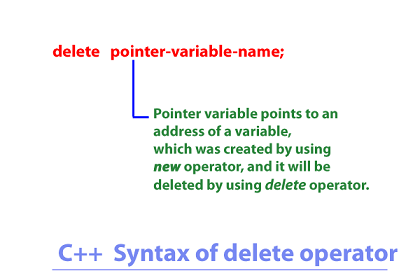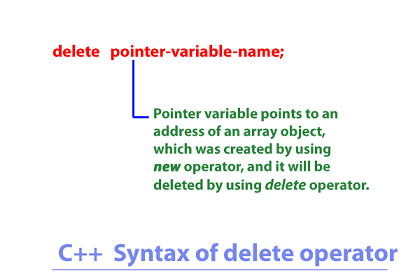< Prev
Next >

C++ delete Operator

C++ provides us two memory management operators which allow us:
• To allocate the memory space for the creation of a new variable or a new object at the runtime, or,
• To free up the memory space which was allocated at the runtime, for the creation of a new variable or an new object.

• These two memory management operators are : new and delete. In our previous article we have already explained the concept of new memory management operator and now it's turn to turn our focus to see how to use the other memory management operator i.e. delete.

• delete operator

• The delete memory management operator allows us free the allocated memory space, which was allocated to a new variable or a new object(an array object) by using the new memory management operator.

There are two syntax of using delete memory management operator depending on what you want to deallocate from the memory i.e. if you are deallocate the memory space of a variable or an object.

delete operator to dellocate a variable.

Let us see how to use the delete operator to free the memory space, which was allocated to a variable by using the new operator.where,
• The pointer-variable-name points to the memory address of a variable whose allocated memory space has to be freed.
• The delete operator is used to deallocate or free the memory space which was allocated to a variable at the runtime by using the new operator.

//C++ Example of delete operator

#include<iostream>

using namespace std;

int main()
{

//Creating and initializing a float variable using new operator in a single line
double *d = new double(101.4);

cout<< "The float value at the address pointed by pointer variable : " << *d << "\n";
cout<< "The memory address allocated to pointer variable : " << d << "\n";

//Freeing the memory space allocated to the double variable by new operator.
delete d;

cout<< "The float value at the address pointed by pointer variable : " << *d << "\n";
cout<< "The memory address allocated to pointer variable : " << d;

return 0;
}

Output

The float value at the address pointed by pointer variable : 101.4
The memory address allocated to pointer variable : 0x16a5c20
The float value at the address pointed by pointer variable : 0
The memory address allocated to pointer variable : 0x16a5c20

• delete operator to deallocate an object(an array object)

• Let us see how to use the delete operator to free the memory space allocated to an object(an array object). The syntax of using delete operator to deallocate an object is going to be just the same as it was for deallocating a variable.where,
• The delete operator is used to deallocate or free the memory space which was previously allocated to an array object at the runtime by using the new operator.
• The pointer-variable-name points to the memory address of an array object whose allocated memory space is about to be freed.

To understand the concept that we have just explained, let us see an example.

//C++ Using delete operator to deallocate an object

#include<iostream>

using namespace std;

int main()
{

//Creating a memory space for a char array with 5 elements, using new operator
char *arr = new char;

arr = 'a';
arr = 'b';
arr = 'c';
arr = 'd';
arr = 'e';

cout<< "The multiple char values in the char array : \n";

for(int i=0;i<5;i++)
{
cout<< arr[i] << "\n";
}

cout<< "The elements contained in an array pointed by pointer variable : " << arr << "\n";

//Deallocating the space allocated to all char elements contained in an array.
delete arr;

//Checking the elements of array after performing delete operation
cout<< "The elements contained in an array after delete operation : " << arr;

return 0;
}

Output

a
b
c
d
e
The elements contained in an array pointed by pointer variable : abcde
The elements contained in an array after delete operation :

• As you can see in the program above, we were able to create the memory space for a char array with 5 elements by using the new keyword.
• Next, we used delete operator to deallocate the memory space allocated to the 5 elements of array.
• Hence, on printing the elements of array after calling the delete operator, does not show the original array elements.